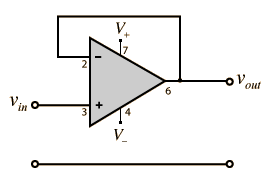Go Down

### Topic: How to calculate voltage drop ? (Read 3106 times)previous topic - next topic##### Jun 01, 2014, 08:52 pmLast Edit: Jun 01, 2014, 10:03 pm by arcadefire Reason: 1
If this is my circuit, how do I calculate voltage drop in the output ?#### JimboZA#1
##### Jun 01, 2014, 08:56 pm
Johannesburg hams call me: ZS6JMB on Highveld rep 145.7875 (-600 & 88.5 tone)

#### polymorph#2
##### Jun 01, 2014, 08:58 pm
You can only calculate it if you have no load drawing current from that. Otherwise, you need to know the load resistance, too.
Steve Greenfield AE7HD
Drawing Schematics: tinyurl.com/23mo9pf - tinyurl.com/o97ysyx - https://tinyurl.com/Technote8

#### wanderson#3
##### Jun 01, 2014, 09:22 pmLast Edit: Jun 01, 2014, 09:40 pm by wanderson Reason: 1
To expand upon the two previous answers.

If your 'load' at that point has a high impedance (resistance), you get a good approximation of the voltage as Vout = (R2 / (R1+R2) * Vin.  Or using your values Vout = (16000 / (25000) * 5 = 3.2V

However, if your 'load' at that point has a low impedance (resistance), say 1000 ohms the numbers change quite a bit since the load is in parallel with R2 (16k).

Two resistors in parallel have a resistence is Rnew = 1 / (1/R1 + 1/R2) = 1 / (0.001 + 0.0000625) = 941 ohms

So your out voltage would be 5V * (941 / 9941) or about 0.5V..   Think I did that math correctly...

'Corrected my error with R1/R1 in the voltage divider formula!

#### JimboZA#4
##### Jun 01, 2014, 09:28 pm
Quote
If your 'load' at that point has a high impedance (resistance), you get a good approximation of the voltage as Vout = (R1 / (R1+R2) * Vin.  Or using your values Vout = (9000 / (25000) * 5 = 1.8V

Should have the 16 on top, not the 9.

But good that you both explained the difference between my assuming it was a "current free" voltage divider and a real load on the output.
Johannesburg hams call me: ZS6JMB on Highveld rep 145.7875 (-600 & 88.5 tone)

#### 68tjs#5
##### Jun 01, 2014, 09:33 pmLast Edit: Jun 01, 2014, 09:36 pm by 68tjs Reason: 1
Ease of calculation depends heavily on the schematic presentation.
There are internationnal standards:
Wherever possible :
-the ground rail is horizontal and placed to the bottom of the graph.
-If there is a power rail it is  horizontal  and placed to the top the graph.
-The inputs are on the left of the schematic.
-The outputs are on the right of the schematic.

Applying this we obtain the diagram attached.
If  the internal resistor of IC connected to the OUT  is very higher than 16 kohms there is no correction to do. Otherwise,  if for example the connected following  IC internal resistor is 50k ohms you must calculate the equivalent resistor:
Re = (*50k* 16k )/ (16k +50 k) = 11.5 k.

Now we consider that  IC internal resistor is very high.
For calculation we will use  Ohms Law U = RI.

We know U = 5V, we know R = (9k + 16k) = 25k
The current is calculated as follows: I = U / R  = 5/25k   I = 0.2 mA
It is now very simple for OUT
OUT = 16k * I
OUT = 0.2 mA*16k  = 3.2 V#6
##### Jun 01, 2014, 09:53 pm
Ok, thank you, one last thing. If I'm using arduinos 5V to power this circuit  (does it have a high impedence, so that Vout = (R2 / (R1+R2) * Vin ?) and if I'm reading the output with arduinos analog pin and than print it (analogRead, Serial.printIn), what values should I get ? I mean... what's the meaning of those values ?

#### wanderson#7
##### Jun 01, 2014, 10:00 pm
It is the impedance/load of what you connect to Out, not the supply voltage from the Arduino.

As a rule, with resistances around 10K (like your example) you are fine with the theorectical value (no need to include load) when connecting the voltage divider to an analog in.  If there is any concern, you can connect an op amp buffer to your out, which presents the out with that ideal high impedance, and provides an ideal 'low impedance' out to whatever device you need to measure the out.

Here is a schematic for a buffer;#8
##### Jun 01, 2014, 10:02 pm
Out goes to an analog pin on arduino#9
##### Jun 01, 2014, 10:15 pm
And if it's a 30K resistance ?

#### wanderson#10
##### Jun 01, 2014, 10:52 pm

And if it's a 30K resistance ?

The analog inputs on the Arduino are reasonably high impedance, so your straight voltage divider isn't going to be much of an issue.  Of course, that is unless you are doing all of the other hoop jumping required to get a accurate voltage reading from the ADC.  Most folks who use the ADC are happy with 'close enough', which is what you see implemented in nearly all Arduino examples.